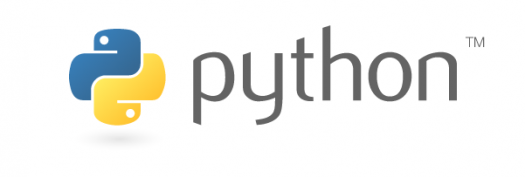# 101 : Test Your Python Skills

10 QuestionsSettings.

• 1.
What is printed by the Python code? print(2 + ‘3')
• A.

23

• B.

5

• C.

Error

• D.

None of these

• 2.
Which of the following is invalid?
• A.

_a = 1

• B.

__a = 1

• C.

__str__ = 1

• D.

None of the mentioned

• 3.
1. What will be the output of the following ?
a = 10         b = 10         print(id(a))         print(id(b))
• A.

Both output will be different

• B.

Both will have the same output

• C.

Cannot say, as it depends on the compiler

• D.

No output, as id() is a void function

• 4.
What does 3 ^ 4 evaluate to?
• A.

81

• B.

12

• C.

0.75

• D.

7

• 5.
What will be the output of the following ? str=“hello" print(str[:2])
• A.

He

• B.

Lo

• C.

Olleh

• D.

Hello

• 6.
What will be the output of the following program? i = 1 while True:     if i%3 == 0:         break     print(i)       i + = 1
• A.

1 2

• B.

1 2 3

• C.

Error

• D.

None of the mentioned

• 7.
Which of the following is a Python tuple?
• A.

[1, 2, 3].

• B.

(1, 2, 3)

• C.

{1, 2, 3}

• D.

{}

• 8.
What will be the output? d = {"john":40, “peter":45} print(d[“john”])
• A.

40

• B.

45

• C.

“john”

• D.

“peter”

• 9.
How many except block can a python try.. except.. block may have
• A.

One

• B.

More than one

• C.

More than zero

• D.

More than Two

• 10.
Which of the below statements is equivalent to add += add ?
• A.

• B.Back to top